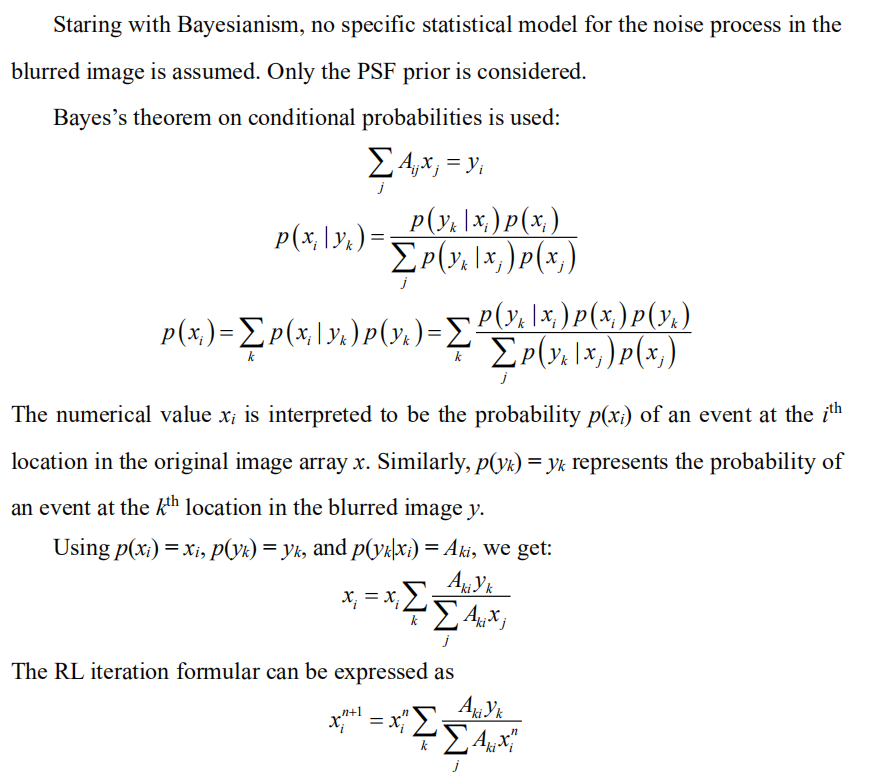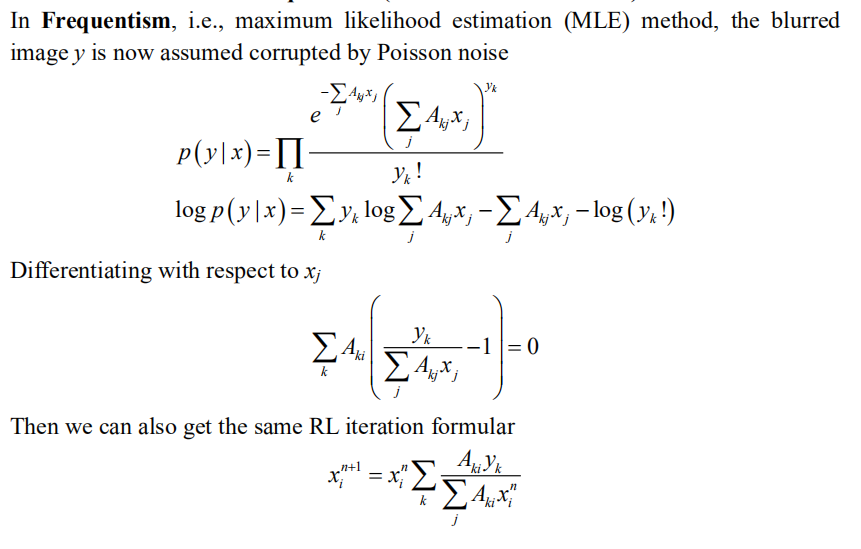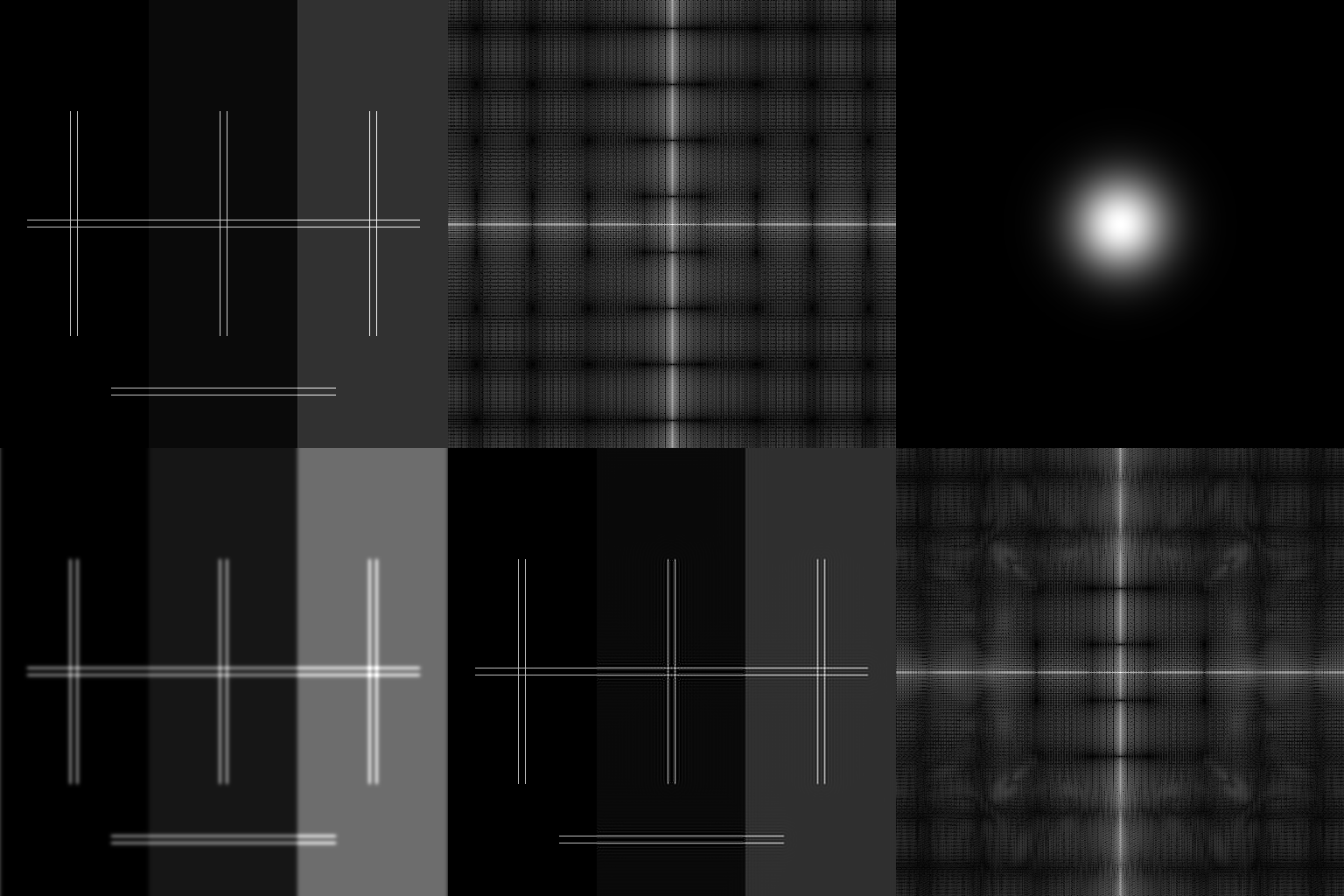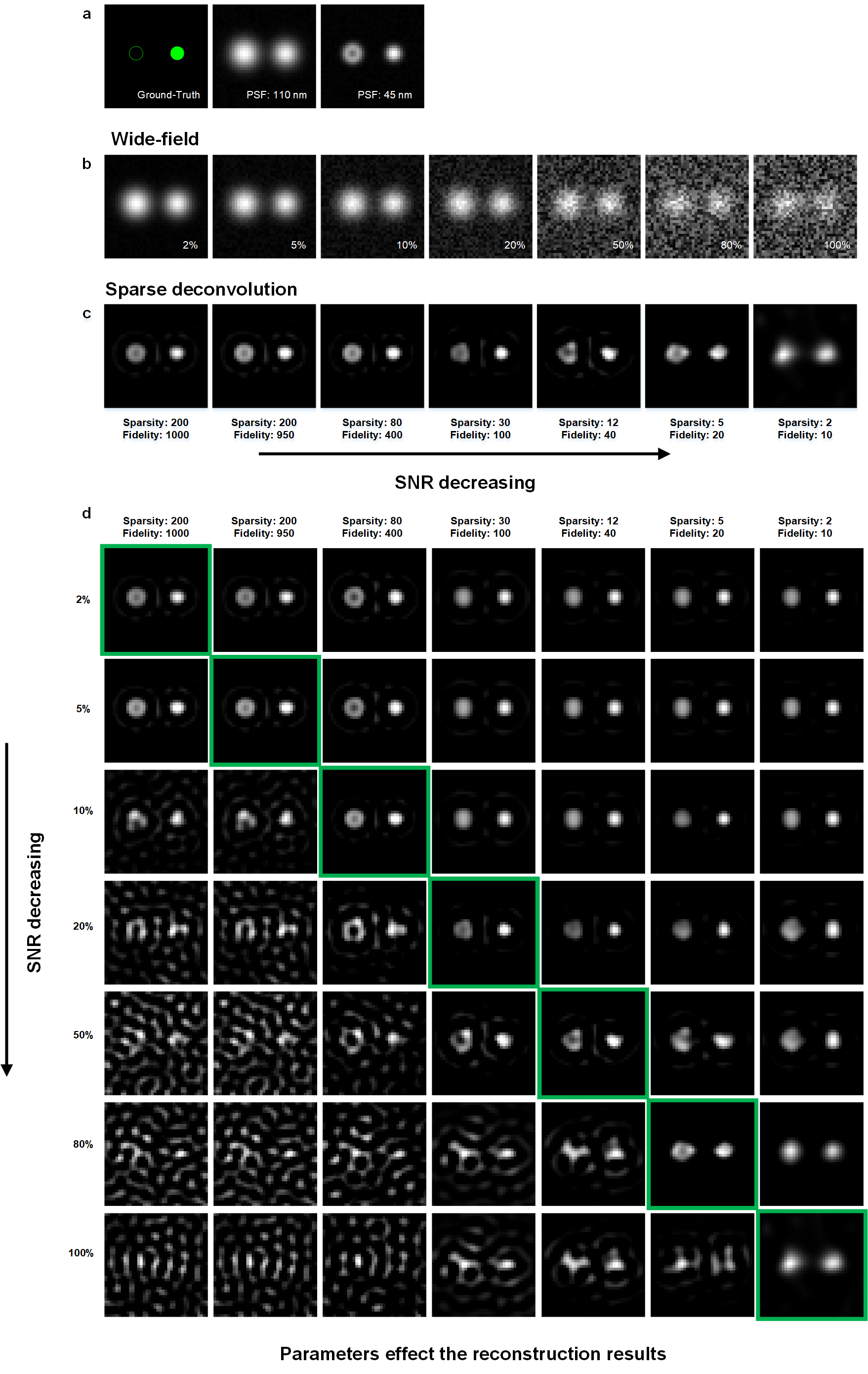rl_positivity_sim

A small blog about deconvolution and machine learning

Words written in the front: Physical resolution is meaningless if in the mathmetical space.

The web version of this blog is recommended.

Inspired by the idea of Andrew for the scientific discussions on GitHub, I post a GitHub page to have an open discussion on the deconvolution topic, all opinions are my own and may not be right.

Inverse problem

First, I intend to talk about a little background of the inverse problem and deconvolution.

For me, the deconvolution is actually a classical machine learning method, rather than an optical method, that estimate the hidden parameter (real signal) from the measured parameter (camera image).

Ax = y. It is an inverse problem if without noise.Ax + n = y. It is an ill-posed inverse problem if with noise.

What we’re doing is trying to estimate the maximum possible x from the observed data y. If the A is a gaussian-function/point-source, and under noise-free condition, the x and y can be one–to–one correspondence.

In the machine learning or convex optimization field, before establishing a model, we always start by asking ourselves, is this problem convex, or is there existing a global optimum. If there is no noise, then the x and y can be one–to–one correspondence. No matter what method we use, we just need to find x that Ax is absolutely equal to y.

There are many solutions, including the Bayesian-based Richardson-Lucy deconvolution, which will be discussed below.

If the computing power is sufficient, even particle swarm (PSO) or genetic algorithm (GA) are effective choices. We can define the x as the parameters to be optimized by GA/PSO, and the optimization will stop when find x for Ax – y = 0.

Frequentism and Bayesianism for the Richardson-Lucy (RL) deconvolution

Lucy’s RL article is the very first successful try on applying machine learning to the optical imaging. However, the logic used in the Lucy’s article is very flexible, so it can be a little misleading in some cases.

What I intend to do in this section is to give a small insight that RL is a branch of classical machine learning method.

The history of RL is very interesting. In fact, for centuries, the Frequentism and the Bayesianism have been at odds. That seems to echo the debate that we have today about the applications of the machine learning on the optical imaging (also in the fields of biology, physics, and chemistry).

To summarize the differences: Frequentism considers probabilities to be related to frequencies of real or hypothetical events. Bayesianism considers probabilities to measure degrees of knowledge. Frequentist analyses generally proceed through use of point estimates and maximum likelihood approaches.

Let’s talk about the RL to show that difference in specific.

Derivation of RL from Bayesianism (one-dimension notation)Derivation of RL from Frequentism (one-dimension notation)The Frequentism and Bayesianism who were all the while “deadly rivals” came together completely at this moment.

Perfect estimation under noise-free condition

In the section of Inverse problem we used the perspective from the field of convex optimization.

What we’re doing is trying to estimate the maximum possible x from the observed data y. If the A is a gaussian-function/point-source, and under noise-free condition, the x and y can be one–to–one correspondence. In the machine learning or convex optimization field, before establishing a model, we always start by asking ourselves, is this problem convex, or is there a global optimal value. If there is no noise, then the x and y can be one–to–one correspondence. No matter what method we use, we just need to find x for Ax is absolutely equal to y. There are many solutions, including the Bayesian-based Richardson-Lucy deconvolution, which will be discussed below. If the computing power is sufficient, even particle swarm (PSO) or genetic algorithm (GA) are effective choices. We can define the x as the parameters to be optimized by GA/PSO, and the optimization will stop when find x for Ax – y = 0.

In addition to the above explanation: the x and y are one–to–one correspondence, or the global optimization existence.

There is another explanation:
The MLE is asymptotically unbiased when the amount of data grows. No noise condition can be considered as collecting infinite number of photons in the field of optical imaging, which is equal to the infinite data, so the MLE is unbiased. In addition, the infinite number of photons will be corresponding to zero variance of estimation. That means the perfect estimation of x.

This should be somehow corresponding to the widely accepted point in the field of single molecule localization.

Small simulations

RL under noise-free condition

Adapted from James’s simulation, we do the RL under noise-free condition for a better illustration.

HERE is the code to generate the following image.It is worth noticing that, the rates of convergence for the areas with and without background are different. More iterations (now is 5k, may be 200k is sufficient) may lead to shorter distance to the ground-truth.

In addition, current RL is a pratical form, e.g., using fast Fourier transform (FFT) instead of convolution in space; replacing near-zero value to a larger one to avoid mistake; accelerated iteration. That will introduce some small corrugate artifacts in the deconvolution results.

Prior knowledge

From a Bayesian perspective, introducing additional reasonable prior knowledge will make the estimation for small data (small photon number, small OTF support) more effective.

Considering the image contained background noise, like the configurations in James’s code, if corresponding to MLE, RL only considers Poisson noise, while Poisson noise does not contain background. So, the RL model seems to be deviating from the range of application (the image with background).

So it would be a good correction/prior-knowledge to add b to the model based on this definition.

Of course, it is extremely complex in real world, including Gaussian-Poisson noise, background noise, defocus signal, cytosol signal, and limited sampling (pixel-size).

The regularization function is necessary for obtaining a unique solution in under-constrained systems.

argmin{L(x, y) + λR(x)},

where x is the reconstructed image, L(·,·) is the data fitting loss between the measured data and the forward model prediction, R(·) is a regularization function on the image, and λ is the hyper-parameter balancing the data fitting loss and the regularization term.

It is always a good way to add the corresponding prior knowledge to power the estimation. This word may be not restricting to deconvolution but all the algorithms.

Taken an example of single molecular localization microscopy (SMLM), which can also be treated as a deconvolution method, it utilizes the strongest prior knowledge on the real fluorescent signal x, i.e., the isolate puncta, to achieve super-resolution in 10~20 times. However, the usage of such prior knowledge needs the specific designed experiment, and can not be applied to the conventional microscopes.

What we intend to do is to find the relatively weak but general (for fluorescence imaging) prior-knowledge in the manuscript,

• PSF is the important prior knowledge, the soul of deconvolution.
• Low frequency background, corresponding to cytosol signal and constant background. This is an optional priori knowledge.

Before moving on to our most important prior knowledge, I intend to say that in the field of machine learning, loss functions are the soul of the methods. This is also the basis for judging whether the prior knowledge is effective. Whether the desired effect [increased resolution in deconvolution] can reduce the loss function with prior knowledge added. If it does, it’s effective for this objective

• Sparsity, we used the L1 norm (the sum of the absolute values of all the elements).

From the forward model of fluorescence imaging, a smaller PSF convolution corresponds to a smaller L1 norm loss function. At least if the forward model of fluorescence imaging is satisfied, then this prior is reasonable.

• Continuity, we used the Hessian matrix norm (the sum of the absolute values of the second order derivative). The notation is [1 -2 1] as in x direction.

The PSF of images must occupy more than 3 × 3 pixels in space which constitutes the basis for the continuity. At least if the Nyquist sampling criteria of images is satisfied, then this prior is reasonable.

Thus, sparsity recovers the high frequency information (towards to the real signal), and in the meantime the image is also constrained by the Hessian matrix continuity. As these priors on two different levels recovering the signal cooperatively, the proposed sparse deconvolution is more robust and effective.

HERE can find more details and results of the sparse deconvolution.

Summary and outlook

Admittedly, the reasonable usage of prior knowledge is a prerequisite for the method to really apply to the biological applications. Unreasonable parameters do lead to less-than-ideal results.

• For example, infinitely increasing the parameter of sparsity will turn the whole picture into zero. The loss function will be equal to the L1 norm only.Simulations under different SNR, and the corresponding reconstruction results. (a) We created synthetic ring-shaped and punctated structures with an 80 nm diameter as ground-truth (left). It was convolved with PSF with FWHM of either 110 nm (middle) or 45 nm (right). (b) These images were subsequently subsampled 16 times (pixel sizes of 16 nm), and corrupted with Poisson noise and 2%, 5%, 10%, 20%, 50%, 80%, and 100% Gaussian noise. (c) The images with different Gaussian noise amplitudes are processed with different weights of priors. (d) The 7 × 7 table of the reconstruction results. The column, and row represent the noise amplitude and weights of priors for reconstruction, respectively.

Although it still has its disadvantages (hard to use), I believe that sparse deconvolution is currently the best and the most effective solution/method. Under a broader perspective, this is probably the TOP method of generative models in the field of unsupervised learning (including deep or non-deep methods) for fluorescence image restoration.

All technologies have its boundaries, and using proof by contradiction to evaluate them may be not appropriate. The success is success, the unsuccessful example will only illustrate the boundary of the technology. Just as all microscopes are suitable for imaging only a certain range of samples.

The unrestricted using and testing can lead to the strange results. But we are eager for the community to test it extensively, and exploring the boundaries of this method, which will also give us or other developers the opportunity to push the algorithm limit further.

Outreach and feedback

FROM Doug Shepherd.

As point out by Doug

The ad hoc choice of parameters is part of what I am confused on. A correct Bayesian treatment would provide error bars and allow you to address the model selection problem that you are side-stepping.
Though it may be a bit off-topic, it is true that deconvolution (MLE, MAP) and feed-forward deep learning approaches for inverse problems typically focus on recovering a point estimate without quantifying the uncertainty (or assuming that the variance is homoscedastic).

The photon number (SNR), sampling size (pixel size), and the OTF size (resolution) will be related to the uncertainty.

As in the field of single molecule localization, the Cram´er Rao Lower Bound (CRLB) was derived to represent the limiting lower bound of the variance for any unbiased parameter estimator. The more photon number, smaller sampling size, and larger OTF, will lead to the smaller uncertainty.

The single molecule localization is actually the simplest situation, and we have already assumed that the estimator is unbiased, which means that the data uncertainty is available and the model error is limited to assess (but the model we established will never be unbiased). If coming to real deconvolution, I thought the assessment of model/data uncertainty will be incredible complicated.

On the other hand, in a Bayesian framework, this problem could be addressed by accurately modeling the measurement noise, formulating an estimation problem, and computing the posterior distribution of the hidden image. However, this analytical approach is only tractable in simple cases. When the inverse problem is non-convex, in high-dimension or the measurement noise is complicated (e.g., non-Gaussian) the posterior distribution can quickly become intractable to compute analytically.

Additionally, in this review, Bayesian statistics and modelling, they propose a new cheklist WAMBS-v2 to correct the model back and forth:

WAMBS-v2, an updated version of the WAMBS (when to Worry and how to Avoid the Misuse of Bayesian Statistics) checklist.

But I thought this checklist may also be not so suitable for the problem in image restoration.

On the other hand, the Bayesian neural networks become popular recently and may be a good choice (the concept) to quantify uncertainty. In addition Bayesian neural networks also split the uncertainty to the model uncertainty and data uncertainty.

The uncertainty assessment is actually an open question, and I thought that there still exists no golden-standard/solution for all cases.

The map has been explored bit by bit, now is indeed a good opportunity for the developers to solve/understand the illed-posed inverse problem futher. By now, the sparse deconvolution still depends on the "human-eye checklist" and I wish that I and my colleagues, and other developers could somehow moderate this imperfection in the future.

Declaration

All opinions are just my own and some content of this blog could be wrong or biased. If someone has a better explanation about this topic #deconvolution, please do let me know using Twitter/GitHub-issue.

 Lucy, L.. “An iterative technique for the rectification of observed distributions.” The Astronomical Journal 79 (1974): 745-754.
 Carasso, A.. “Linear and Nonlinear Image Deblurring: A Documented Study.” SIAM Journal on Numerical Analysis 36 (1999): 1659-1689.
 VanderPlas, Jake. “Frequentism and bayesianism: a python-driven primer.” arXiv preprint arXiv:1411.5018 (2014).
 Zhao, Weisong, et al. “Extending resolution of structured illumination microscopy with sparse deconvolution.” (2021).